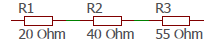# Resistors in Series

Description how to calculate resistors in series

## Resistors in Series

When multiple resistors are connected in a line where the current flows through them in sequence, we call it a series connection of resistors.### Total resistance

The total resistance results from the addition of the individual resistors

$$R_{ges} = R_1+R_2+R3$$

### Current

The flowing current through the individual resistors is equal and corresponds to the total current of the circuit.

$$I_{ges}=R_1+R_2+R_3$$
$$\displaystyle I=\frac{U}{R_{ges}}$$

### Voltage

The total voltage across the total resistance is the sum of the individual voltages at the individual resistors

$$U=U_1+U_2+U_3$$

The applied total voltage is divided by the individual resistances in proportion to their values.

$$\displaystyle \frac{U_{ges}}{R_{ges}}=\frac{U_1}{R_1}=\frac{U_2}{R_2}=\frac{U_3}{R_3}$$

From this, the formula for a single voltage can be derived.

$$\displaystyle U_1=\frac{R_1·U_{ges}}{R_{ges}}$$

### Example

We calculate the total resistance, the current and the individual voltages at the resistors. The total voltage is given with $$230$$ Volt.$$\displaystyle R_{ges}=R_1+R_2+R_3=20+40+55=115Ω$$
$$\displaystyle I=\frac{U}{R_{ges}}=\frac{230}{115}=2A$$
$$\displaystyle U_1=R_1·I=20·2=40V$$
oder

$$\displaystyle U_1=\frac{R_1·U_{ges}}{R_{ges}}=\frac{20·230}{115}=40V$$
$$\displaystyle U_2=R_2·I=40·2=80V$$
oder

$$\displaystyle U_2=\frac{R_2·U_{ges}}{R_{ges}}=\frac{40·230}{115}=80V$$
$$\displaystyle U_3=R_3·I=55·2=110V$$
oder

$$\displaystyle U_3=\frac{R_3·U_{ges}}{R_{ges}}=\frac{55·230}{115}=110V$$